# Morris Preorder Tree Traversal in C++

In this tutorial, we will learn how to implement Morris preorder tree traversal in C++. Before that let’s understand what is preorder tree traversal.

Preorder Tree Traversal: It is a type of tree traversal in which first we visit Root then Left subtree and then Right Subtree of the graph.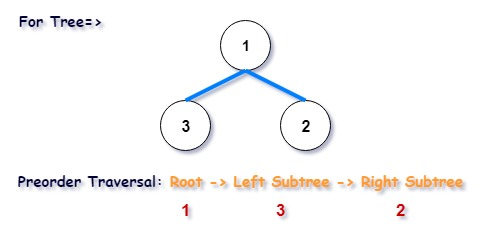## Steps of Morris Preorder Tree Traversal algorithm:

Step 1:

If root->left==NULL {
print root->data
root=root->right
}
else{

Find preorder predecessor

Make preorder predecessor’s right child point to the curr node.

If the right child of the preorder predecessor is already pointing to the curr node{

Set the right child to NULL.

Move to the right child of the curr node.

}
else{

Set it to the curr node.

Print the data of curr node and move to the left child of the curr node.

}
}

Step 2:

Repeat step 1 until the root becomes NULL.

## Flow of Morris Preorder Tree Traversal

Input tree: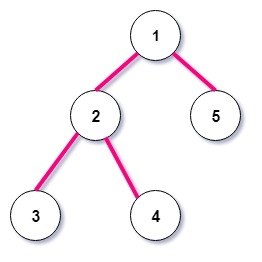Iteration 1: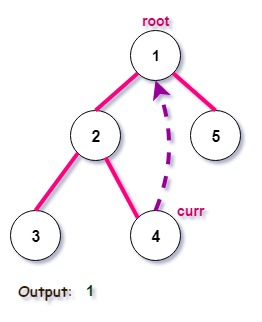Iteration 2:Iteration 3: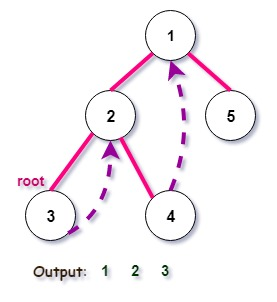Iteration 4:Iteration 5:Iteration 6: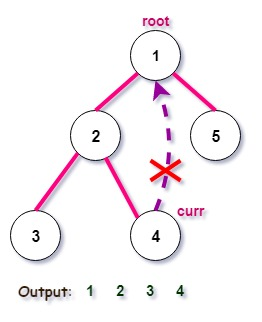Iteration 7: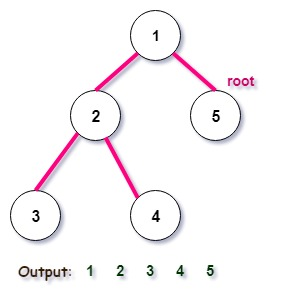## Program to implement Morris Preorder Tree Traversal in C++

```#include<iostream>
using namespace std;

/* Each node of a tree consists of a 1 data field and 2 links, 1 for left child and 1 for right child */
typedef struct Node
{
int data;
Node* left;
Node* right;
}Node;

Node* new_node(int );
void morris_preorder_traversal(Node*);
Node* find_preorder_predecessor(Node*);

int main()
{
Node* root = new_node(1);
root->left = new_node(2);
root->right = new_node(5);
root->left->left = new_node(3);
root->left->right = new_node(4);
/* this input tree is shown in above figure */

cout<<"Morris Preorder Traversal of the graph: ";
morris_preorder_traversal(root);

return 0;
}

/* This function is used to create new node with the given data */
Node* new_node(int data)
{
Node* node = new Node;
node->data = data;
node->left = NULL;
node->right = NULL;
return node;
}

Node* find_preorder_predecessor(Node* root)
{
Node* curr=root->left;
while (curr->right && curr->right != root)
curr=curr->right;

return curr;
}

void morris_preorder_traversal(Node* root)
{
while (root)
{
if (root->left==NULL)
{
cout<<root->data<<" ";
root=root->right;
}
else
{
/* Finding preorder predecessor */
Node* curr=find_preorder_predecessor(root);

/* If preorder predecessor's right child is already pointing to root */
if (curr->right == root)
{
curr->right = NULL;
root=root->right;
}
else
{
cout<<root->data<<" ";
curr->right = root;
root = root->left;
}
}
}
}
```

Output:

`Morris Preorder Traversal of the graph: 1 2 3 4 5`

#### Time Complexity & Space Complexity

The Time Complexity and Space Complexity of the program is O(n) and O(1) respectively.